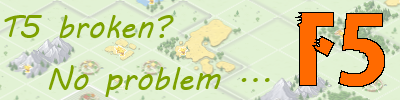# Crop from stolen goods

• Hey guys,

I have tried to find it here in the forum, but did not get a satisfactory answer to my question:

What is the calculation formula for the crops in stolen goods? I know it is 1 crop per stolen good for the first 4k treasures in the kingdom, and I know it gradually becomes les afterwards. But does anyone have the formula? From what I observed, it is a partially defined function, such as

1:1 for the first 4k
1:2 for 4-8k
1:3 for 8-12k

and so on. Anyone knows for sure?

• 1 : 1 for the first 4k is correct, after that TG doesn't tell us anything and I never collected enough data points to inspect the formula further.A "formula" similar to yours had kinda close results to 1 or 2 random points I tested it with, but that doesn't mean anything at all. Unfortunately, the amount is calculated serverside and the formula isn't anywhere in the client side source code. :c

If someone did the work and collect a big amount of data points and analyzed it, I'd appreciate a formula aswell. xD• I am quite sure it is a partially defined function. I also think there is a linear relationship in each of the function's segments, so if T is the amount of treasures and C the amount of crop, the function is something like:

for T <= 4k: C = T
for 4k< T <= X1: C = 4000 + (T-4000) * (Y1-4000)/(X1-4000)

for X1 < T <= X2: C = Y1 + (T-X1)* (Y2-Y1)/(X2-X1)

...

This gives us roughly twice as many variables as functions, hence not possible to solve from empirical dataBut maybe, with enough data points, it would be possible to define an approximation function?

• Yeah, I played around with something like that, too.But like I said, I never gathered enough data to really analyze it deeper.• https://www.desmos.com/calculator/safahqcgkm

it is 50 crop off.you can click on graph and move to see the crop amounts.my biggest sample was "160k treasures : 11k crop", so beyond 160k I'm not sure how it behaves.

• At 204,973 treasures I see 12,400 crop. Not sure if that helps your data.

• Age of server is a key factor in crop bonusBM

• At 204,973 treasures I see 12,400 crop. Not sure if that helps your data.

Thanks!

formula is the same, just increase the limit (range):

• Thanks!

formula is the same, just increase the limit (range):I guess the limits are potentials of 2, so it should be 32,000 64,000 128,000 256,000

But that somehow does not work: 32k reach until 7k crop, 64k until 8k crop, 128k until 9k crop and 256k should then go to 10k crop. But Renuo said 200k treasure make fo 12400 crop. So maybe the limits are less sparse for higher numbers? Such as 32k, 50k, 75k, 100k, 150k, 200k? Because then it works!

• I guess the limits are potentials of 2, so it should be 32,000 64,000 128,000 256,000

But that somehow does not work: 32k reach until 7k crop, 64k until 8k crop, 128k until 9k crop and 256k should then go to 10k crop. But Renuo said 200k treasure make fo 12400 crop. So maybe the limits are less sparse for higher numbers? Such as 32k, 50k, 75k, 100k, 150k, 200k? Because then it works!

no

after 32K treasures there is no change in slope, my samples from game just fits in 5th formula

so I guess from 32k to infinity (at least 205k), you will get extra 50 crop per 1600 treasures.

• no

after 32K treasures there is no change in slope, my samples from game just fits in 5th formula

so I guess from 32k to infinity (at least 205k), you will get extra 50 crop per 1600 treasures.

Ahh sorry, then I missunderstood you. Yes, the numbers Renuo and Jallu gave fit into your formula! So likely you are right• Age of server is a key factor in crop bonus

Hmm, how would that be fit into lua's formula? His numbers seem to be right. From what I know (credits to Be2-e4) is that the non-crop bonus is somehow related to server age. More precisely, it is related to top player income.